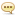Tutorials :

Udemy - Matrices & Determinants

Author: Baturi   |   04 December 2021   |: 0MP4 | Video: h264, 1280x720 | Audio: AAC, 44.1 KHz
Language: English | Size: 2.18 GB | Duration: 1h 55m
Applications of Matrices & Determinants

What you'll learn
Concepts of Matrices & Determinants
Requirements
No
Description
A matrix is a arrangement of numbers in rows and columns. Matrices may be rectangular array or square array. Matrices are useful in a variety of fields and form the basis for linear algebra. Generally, in a matrix, the vertical elements are termed as columns and the horizontal elements are termed as rows. The order of a matrix is measured in the number of rows and columns the matrix has. The above matrix, for instance, has 2 rows and 3 columns, and thus it is of order 2x3 matrix. Matrices that have the same number of rows as columns are called square matrices and are of particular interest. Their applications include solving systems of linear equations, path-finding in graph theory, and several applications in group theory (especially representation theory). They are also extremely useful in representing linear transformations and row operations. oncept in the field of linear algebra. The subject of matrices has been researched and expanded by the works of many researchers and prominent mathematicians, who have found numerous applications of matrices in various disciplines such as Economics, Engineering, Statistics and various other sciences. In this course, the following applications to matrices will be discussed:
Basic Types of Matrices
Applications of Matrix Addition and Subtraction
Applications of Multiplication of Matrices
Applications of System of Linear Equations using Cramers Rule and Inverse Method
Leontief Input-Output Model
Gauss Jordan Model
Who this course is for:
Graduation Students, Management Students, MBA, BBA, Commerce students, Data Analytics students

Homepage
https://www.udemy.com/course/matrices-determinants/